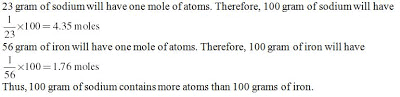Courses

# Chapter Questions - Atoms and Molecules, Class 9, Science Class 9 Notes | EduRev

## Class 9 : Chapter Questions - Atoms and Molecules, Class 9, Science Class 9 Notes | EduRev

The document Chapter Questions - Atoms and Molecules, Class 9, Science Class 9 Notes | EduRev is a part of Class 9 category.
All you need of Class 9 at this link: Class 9

### Chapter 3: Atoms and Molecules

Questions, Page 32 – 33

1. In a reaction, 5.3 g of sodium carbonate reacted with 6 g of ethanoic acid. The products were 2.2 g of carbon dioxide, 0.9 g water and 8.2 g of sodium ethanoate. Show that these observations are in agreement with the law of conservation of mass. sodium carbonate + ethanoic acid → sodium ethanoate + carbon dioxide + water
Solution:
The reactants in this reaction are sodium carbonate and the ethanoic acid and the products are carbon dioxide, water and sodium ethanoate.
The total mass of the reactants = 5.3 + 6 = 11.3 g
The total mass of the products = 2.2 + 0.9 + 8.2 = 11.3 g
Thus, the total mass of the reactants is same as that of the products. Thus, the mass was neither created nor destroyed during the chemical reaction. Therefore, the mass was conserved during the reaction of sodium carbonate and ethanoic acid.

2. Hydrogen and oxygen combine in the ratio of 1:8 by mass to form water. What mass of oxygen gas would be required to react completely with 3 g of hydrogen gas?
Solution: The law of constant proportions states that in a chemical substance the elements are always present in definite proportions by mass. Hydrogen and oxygen combine in the ratio of 1:8 by mass to form water. Thus, in order to form water, 3 × 8 = 24 g of oxygen will react completely with 3 g of hydrogen gas.

3. Which postulate of Dalton’s atomic theory is the result of the law of conservation of mass? Solution: The second postulate of Dalton’s atomic theory which states that “Atoms are indivisible particles, which cannot be created or destroyed in a chemical reaction” is a result of the law of conservation of mass.

4. Which postulate of Dalton’s atomic theory can explain the law of definite proportions? Solution: The sixth postulate of Dalton’s atomic theory which states that “The relative number and kinds of atoms are constant in a given compound” can explain the law of definite proportions.

Questions, Page 34

1. Define the atomic mass unit.
Solution: Atomic mass unit is a mass unit equal to exactly one-twelfth the mass of a carbon-12 atom. It is used to measure the masses of subatomic particles.

2. Why is it not possible to see an atom with naked eyes?
Solution:
The dimension of atoms is very small of the order of 1 nm. More than a million atoms when staked one over the other will make a layer that is barely as thick as a sheet of paper. Thus, we cannot see an atom with naked eyes.

Questions, Page 39

1. Write down the formulae of
(i) sodium oxide
(ii) aluminium chloride
(iii) sodium suphide
(iv) magnesium hydroxide
Solution:
(i) Na2O
(ii) AlCl3
(iii) Na2S
(iv) Mg(OH)2

2. Write down the names of compounds represented by the following formulae:
(i) Al2(SO4)3
(ii) CaCl2
(iii) K2SO4
(iv) KNO3
(v) CaCO3.
Solution:
(i) Aluminium Sulphate
(ii) Calcium chloride
(iii) Potassium sulphate
(iv) Potassium nitrate
(v) Calcium carbonate

3. What is meant by the term chemical formula?
Solution: The chemical formula of a compound is a symbolic representation of its composition.

4. How many atoms are present in a
(i) H2S molecule and
(ii) PO43– ion?
Solution:
(i) Three (two hydrogen atoms and one sulphur atom)
(ii) Five (One phosphorus and four oxygen)

Questions, Page 40

1. Calculate the molecular masses of H2, O2, Cl2, CO2, CH4, C2H6, C2H4, NH3, CH3OH.
Solution:
Molecular mass of H2 = 2u
Molecular mass of O2 = 16u + 16u = 32u
Molecular mass of Cl2 = 35.5u + 35.5u = 71u
Molecular mass of CO2 = 12u + (2 × 16u) = 44u
Molecular mass of CH4 = 12u + (4 × u) = 16u
Molecular mass of NH3 = 14u + (3 × u) = 17u
Molecular mass of CH3OH = 12u + (3 × u) + 16u + u = 32u

2. Calculate the formula unit masses of ZnO, Na2O, K2CO3, given atomic masses of Zn = 65u, Na = 23u, K = 39u, C = 12u, and O = 16u.
Solution:
Formula unit mass of ZnO = 65u + 16u = 81u
Formula unit mass of Na2O = (2 × 23u) + 16u = 66u
Formula unit mass of K2CO3 = (2 × 39u) + 12u + (3 × 16u) = 138u

Questions, Page 42

1. If one mole of carbon atoms weighs 12 gram, what is the mass (in gram) of 1 atom of carbon?
Solution: One mole of carbon atoms weighs 12 gram i.e., 6.022 × 1023 atoms of carbon weigh 12 gram. Therefore, 1 atom of carbon weighs 12 ÷ (6.022 × 1023) = 1.993 × 10–23 gram.

2. Which has more number of atoms, 100 grams of sodium or 100 grams of iron (given, atomic mass of Na = 23 u, Fe = 56 u)?
Solution:Offer running on EduRev: Apply code STAYHOME200 to get INR 200 off on our premium plan EduRev Infinity!

,

,

,

,

,

,

,

,

,

,

,

,

,

,

,

,

,

,

,

,

,

,

,

,

,

,

,

;